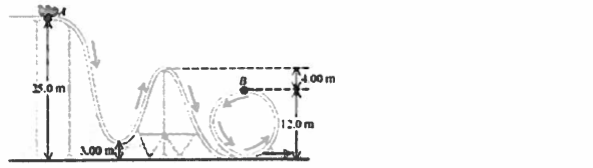Problem: A 240 kg roller coaster car starts from rest at point A and slides down the frictionless loop-the-loop shown in the accompanying figure. Point A is 25.0 m above the ground and point B is 12.0 m above the ground. The height of other points on the track are shown in the diagram.How fast is this roller coaster moving at point B?

FREE Expert Solution
89% (366 ratings)
Problem Details

A 240 kg roller coaster car starts from rest at point A and slides down the frictionless loop-the-loop shown in the accompanying figure. Point A is 25.0 m above the ground and point B is 12.0 m above the ground. The height of other points on the track are shown in the diagram.

How fast is this roller coaster moving at point B?